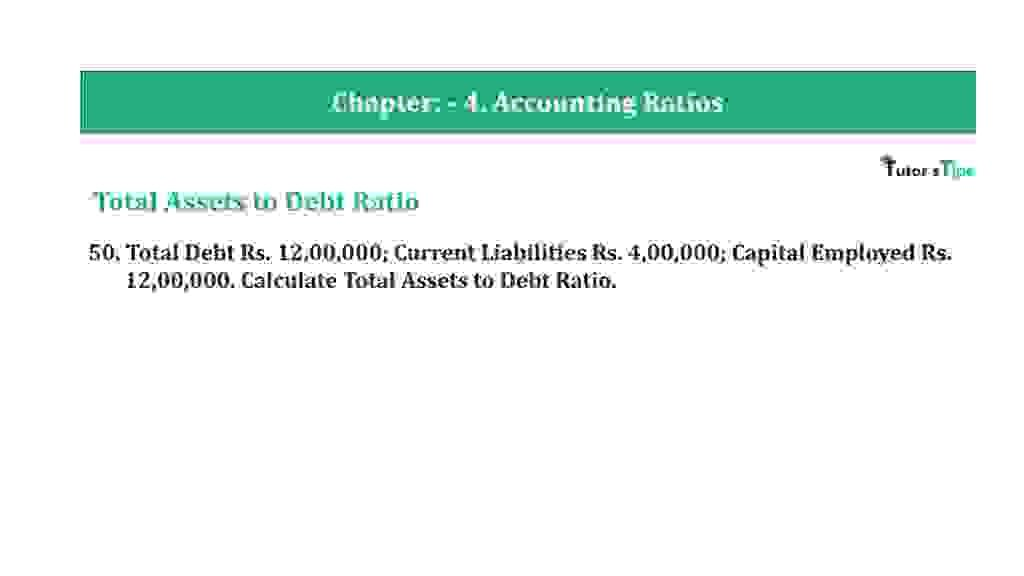# Question 50 Chapter 4 of +2-B – T.S. Grewal 12 ClassQuestion 50 Chapter 4 of +2-B

Total Assets to Debt Ratio

50. Total Debt Rs. 12,00,000; Current Liabilities Rs. 4,00,000; Capital Employed Rs.12,00,000. Calculate Total Assets to Debt Ratio.

### The solution of Question 50 Chapter 4 of +2-B: –

 Total Debts = Rs. 12,00,000 Working Capital = Current Asset – Current Liabilities Current Liabilities = Working Capital – Current Assets = Rs. 5,00,000 – Rs. 1,00,000 Current Liabilities = Rs. 4,00,000 Debt = Total Debts – Current Liabilities = Rs. 12,00,000 – Rs. 4,00,000 Debt = Rs. 8,00,000 Total Assets = Shareholders’ Funds + Total Debt = Rs. 2,00,000 – Rs. 12,00,000 Total Assets = Rs. 14,00,000

 Total Assets to Debt Ratio = Total Assets = Rs.14,00,000 Long- term Debts Rs. 8,00,00 = 1.75: 1

Balance Sheet: Meaning, Format & Examples

Thanks, Please Like and share with your friends

Comment if you have any question.

Also, Check out the solved question of previous Chapters: –

### T.S. Grewal’s Double Entry Book Keeping (Vol. II: Accounting for Companies)T.S. Grewal’s Analysis of Financial Statements